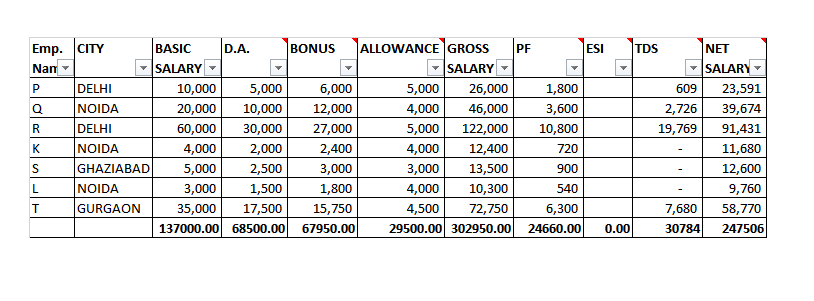Count Formulas

Accounts Tax
Excel

Instead of Filters,we can also use Countif Formula

Formula of Countif

=countif(Select Area,"Write Criteria")

Note:-

Criteria here is always in inverted commas "    "

#### Assignment

Resolve last question using countif and countifsQ1 COUNT THE NUMBER OF DELHI EMPLOYEES

Q2 COUNT THE NUMBER OF NOIDA EMPLOYEES

Q3 COUNT THE NUMBER OF EMPLOYEES GETTING BASIC > 20000?

Q4 COUNT THE NUMBER OF EMPLOYEES GETTING BONUS < 5000?

Q5 COUNT THE NUMBER OF EMPLOYEES PAYING ESI?

Q6 COUNT THE NUMBER OF EMPLOYEES WHOSE TDS DEDUCTED?

Q7 COUNT THE NUMBER OF EMPLOYEES WHOSE TDS DEDUCTED >1000?

Q8 COUNT THE NUMBER OF DELHI EMPLOYEES GETTING BASIC >20000?

Q9 COUNT THE NUMBER OF DELHI EMPLOYEES GETTING BONUS <5000?

Q10 COUNT THE NUMBER OF DELHI EMPLOYEES GETTING BASIC >20000 AND BONUS <5000?

Q11 COUNT THE NUMBER OF NOIDA EMPLOYEES PAYING ESI?

Q12 COUNT THE NUMBER OF NOIDA EMPLOYEES PAYING ESI AND GETTING BONUS>2000?

Note

If there are more than one condition,we use Countifs instead of Countif

Formula of Countifs

=countifs(Select Area 1,"Write Criteria 1",Select Area 2,"Write Criteria 2")

DIFFERENCE BETWEEN COUNTIF AND COUNTIFS

 COUNTIF COUNTIFS it is used if there is only one condition It is used if there is 2 or more conditions Formula of Countif =countif(Select Area,"Write Criteria") Formula of Countifs =countifs(Select Area 1,"Write Criteria 1",Select Area 2,"Write Criteria 2")

Get live Maths 1-on-1 Classs - Class 6 to 12